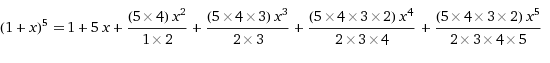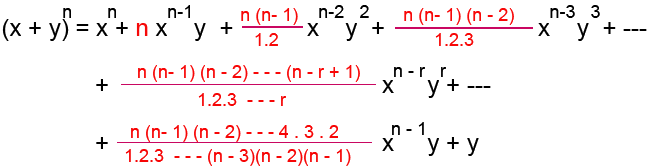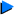# The Binomial Theorem

Observe the following expansion.

(1 + x)5 = 1 + 5x + 10x2 + 10x3 + 5x4 + x5

This expression can also be expressed as follows:In general,if n is a positive integer,This expression is known as the Binomial Theorem.

Where the general coefficient or the coefficient of (r + 1)th term is denoted as follows:Note:
For the expansion of (x + y)
n
,where n is a positive integer,

(i) the binomial coefficients are all integers.
(ii) nC0 =nCn =1, nCn =nCn-1 = n, nCr =nCn-r .
The general term or the (r + 1)th term of the expansion of (x + y)n is determined as follows:

(r + 1)th term = nCr xn-r yr

Example 1
If the coefficients of x4 and x5 in the expansion of (3 + kx)10 are equal, find the value k.
(3 + kx)10 ကိုျဖန္႔္လွ်င္ x4 ၏ေျမွွာက္ေဖၚကိန္းသည္ x5 ၏ေျမွွာက္ေဖၚကိန္းႏွင့္ တူညီလွ်င္ k ၏တန္ဖိုးကို ရွာပါ။Solution
(3 + kx)10
(r + 1)th term = 10Cr 310-r (kx)r = 10Cr 310-r krxr

Here
10Cr 310-r kr is the coefficient of xr.
coefficient of x4 = 10C4 36 k4
coefficient ofx5 = 10C5 35 k5

By the problem,
10C4 36 k4 = 10C5 35 k4
10C4 36 k4 = 10C4 (6/5) 35 k5
3 = (6/5)k
k = 5/2

Example 2
I
n the expansion of,a x7 is four times the coefficient of x10. Find the value a.ကိုျဖန္႔္လွ်င္ x7 ၏ေျမွွာက္ေဖၚကိန္းသည္ x10 ၏ေျမွွာက္ေဖၚကိန္း၏ 4 ဆျဖစ္လွ်င္ a ၏တန္ဖိုးကို ရွာပါ။Solution(r + 1)th term =8Cr arx16-3r
To get the coefficient of x7, 16-3r = 7 and r =3
To get the coefficient of x10, 16-3r = 10 and r =2

Therefore,
coefficient of x7 = 8C3 a3
coefficient of x10 = 8C2 a2

By the problem,
8C3 a3 = 4 8C2 a2
8C2 (6/3) a3 = 4 8C2 a2
a = 2